# The first person to understand that an object's natural motion may be one of rest or...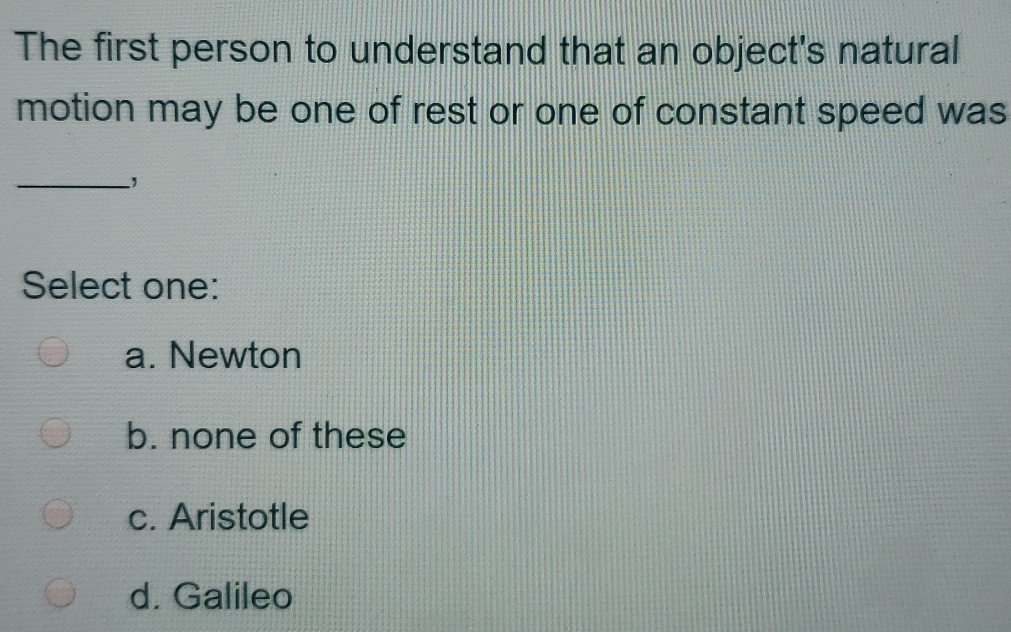The first person to understand that an object's natural motion may be one of rest or one of constant speed was Select one: a. Newton b. none of these c. Aristotle d. Galileo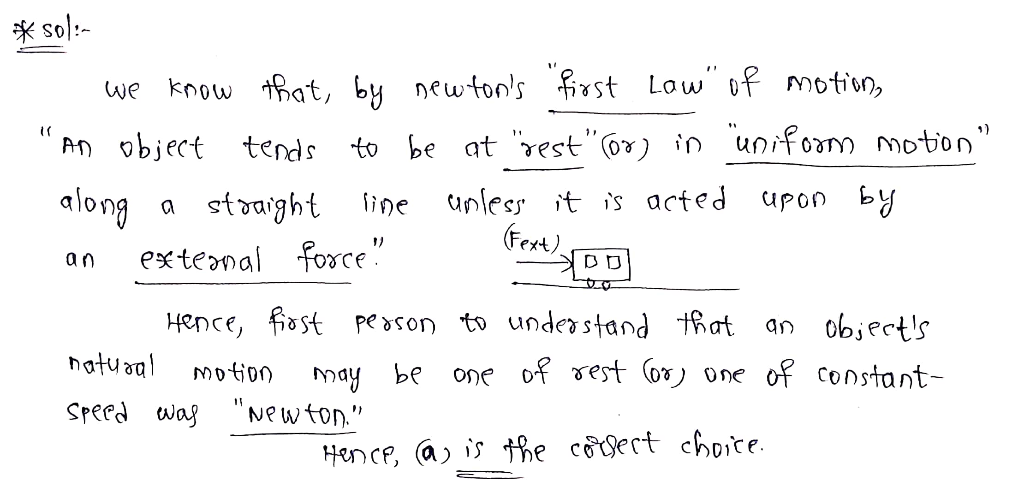#### Earn Coin

Coins can be redeemed for fabulous gifts.

Similar Homework Help Questions
• ### The first major failure of the Ptolemaic Theory to predict the results of observations was O...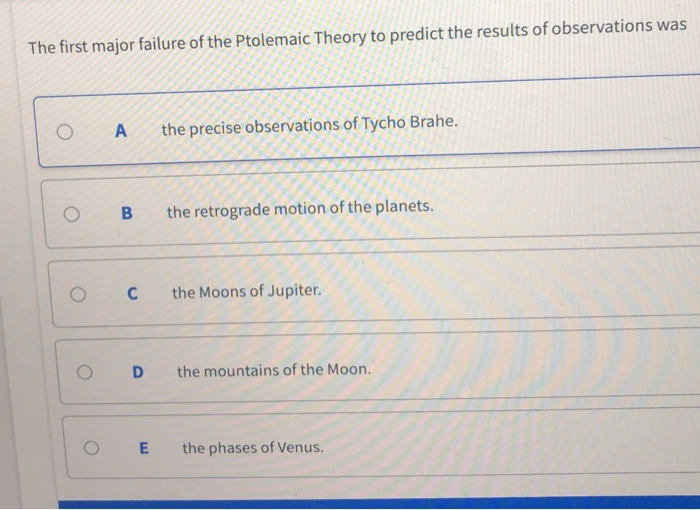The first major failure of the Ptolemaic Theory to predict the results of observations was O Athe precise observations of Tycho Brahe O B the retrograde motion of the planets. C the Moons of Jupiter. O D the mountains of the Moon. OE the phases of Venus. The first telescope was (possibly) built by O A Galileo O B Newton. Oc Someone in the Netherlands. O D Tycho Brahe. Aristotle said that the amount of force on a moving earthly...

• ### 1] As a projectile is flying (following the standard projectile motion seen in class), it lodes...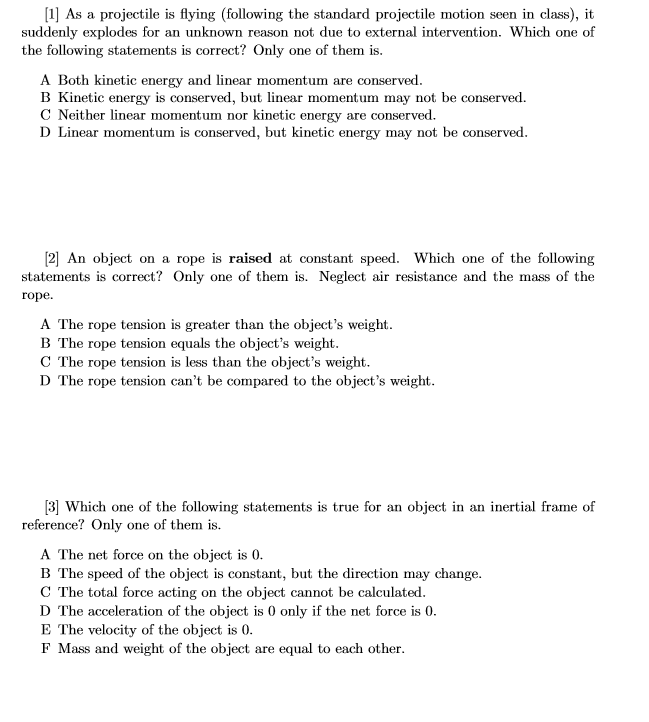1] As a projectile is flying (following the standard projectile motion seen in class), it lodes for an unknown reason not due to external intervention. Which one of suddenly exp the following statements is correct? Only one of them is. A Both kinetic energy and linear momentum are conserved B Kinetic energy is conserved, but linear momentum may not be conserved. C Neither linear momentum nor kinetic energy are conserved. D Linear momentum is conserved, but kinetic energy may not...

• ### what is considered rational by one person may not be rational to another person because What...what is considered rational by one person may not be rational to another person because What is considered "rational" by one person may not be "rational" to another person because Select one: a. Of ethical considerations that give rise to different value judgments. b. People have different preferences. C. Of the effects on the decision rendered by considering the future. d. Some people may have access to more information than others. e. All of the above.

• ### Based on what you know about falling objects, select all of the correct statements from the...

Based on what you know about falling objects, select all of the correct statements from the following list. (Ignore the effects of air resistance.) a. The speed of a falling object depends on its weight. b. Objects tend to slow down naturally unless propelled by a force. c. According to tradition, Galileo dropped objects of different mass from the leaning tower of Pisa. d. Inertia is an object's tendency to resist changes in its motion. e. The speed of falling...

• ### A rigid object, hinged at one end and initially at rest, is set into rotational motion....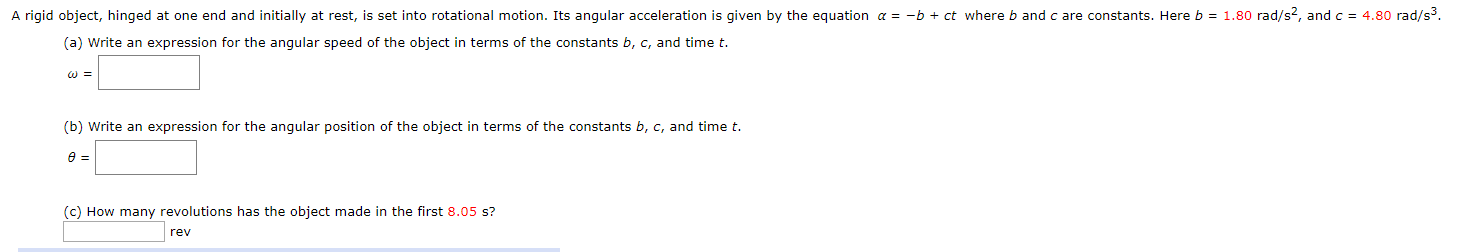A rigid object, hinged at one end and initially at rest, is set into rotational motion. Its angular acceleration is given by the equation a = -b + ct where band care constants. Here b = 1.80 rad/s2, and c = 4.80 rad/s3. (a) Write an expression for the angular speed of the object in terms of the constants b, c, and time t. W = (b) Write an expression for the angular position of the object in terms of...

• ### Motion diagram question 19. For the following motion diagrams, draw an arrow to indicate the displacement...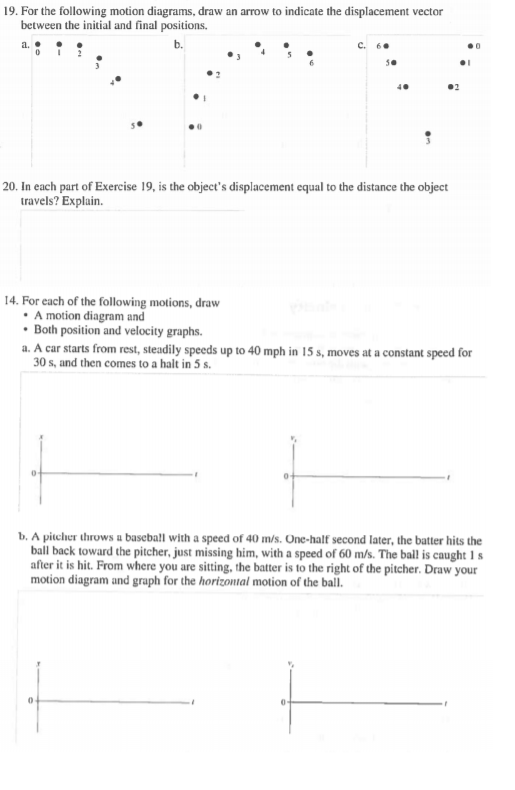Motion diagram question 19. For the following motion diagrams, draw an arrow to indicate the displacement vector between the initial and final positions. b. 40 20. In each part of Exercise 19, is the object's displacement equal to the distance the object travels? Explain. 14. For each of the following motions, draw A motion diagram and Both position and velocity graphs a. A car starts from rest, steadily speeds up to 40 mph in 15 s, moves at a constant...

• ### A rigid object, hinged at one end and initially at rest, is set into rotational motion....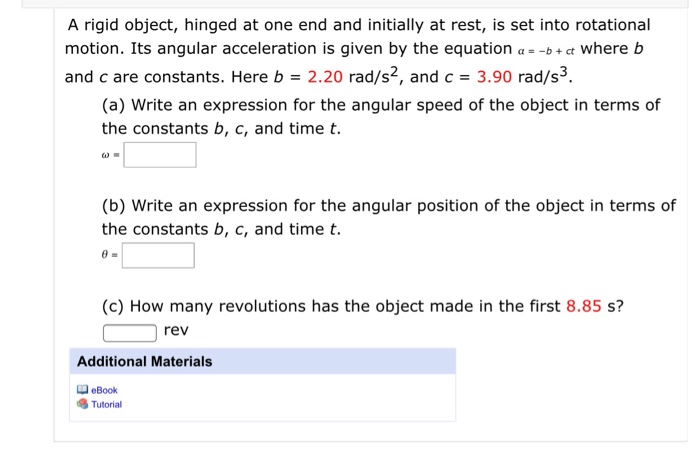A rigid object, hinged at one end and initially at rest, is set into rotational motion. Its angular acceleration is given by the equation a = -b + ct where b and c are constants. Here b = 2.20 rad/s2, and c = 3.90 rad/s3. (a) Write an expression for the angular speed of the object in terms of the constants b, c, and time t. (b) Write an expression for the angular position of the object in terms of...

• ### An object's motion is represented by the x vs. t graph shown below Hint: Velocity is...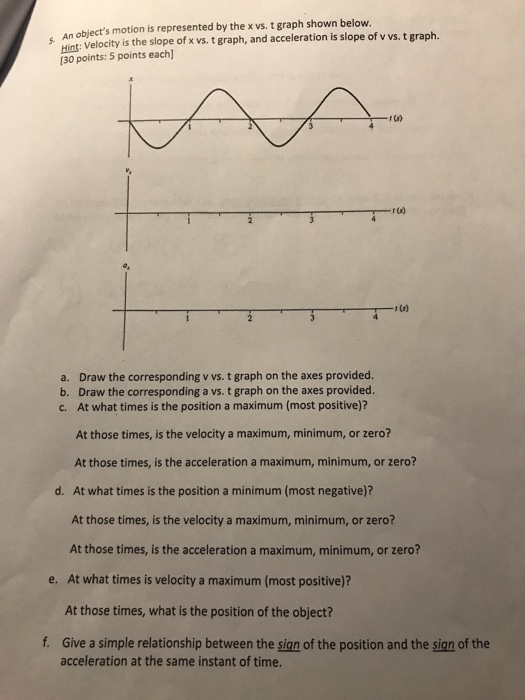An object's motion is represented by the x vs. t graph shown below Hint: Velocity is the slope of x vs. t graph, and acceleration is slope of v vs. t graph. 130 points: 5 points each r (s) t (a) a. Draw the corresponding v vs. t graph on the axes provided. b. Draw the corresponding a vs. t graph on the axes provided. c. At what times is the position a maximum (most positive)? At those times, is...

• ### aestion 2 When a person stands on a scale in an elevator at rest, the scale...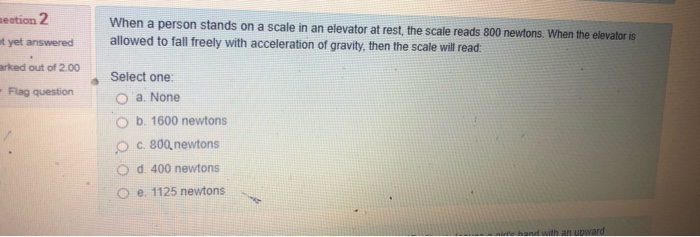aestion 2 When a person stands on a scale in an elevator at rest, the scale reads 800 newtons. When the elevator is allowed to fall freely with acceleration of gravity, then the scale will read: ot yet answered arked out of 2.00 - Flag question Select one: О'a None b. 1600 newtons O c. 800, newtons O d. 400 newtons e. 1125 newtons hand with an upward

• ### 2. The following velocity-vs-time graph represents the motion of a race car during the first few...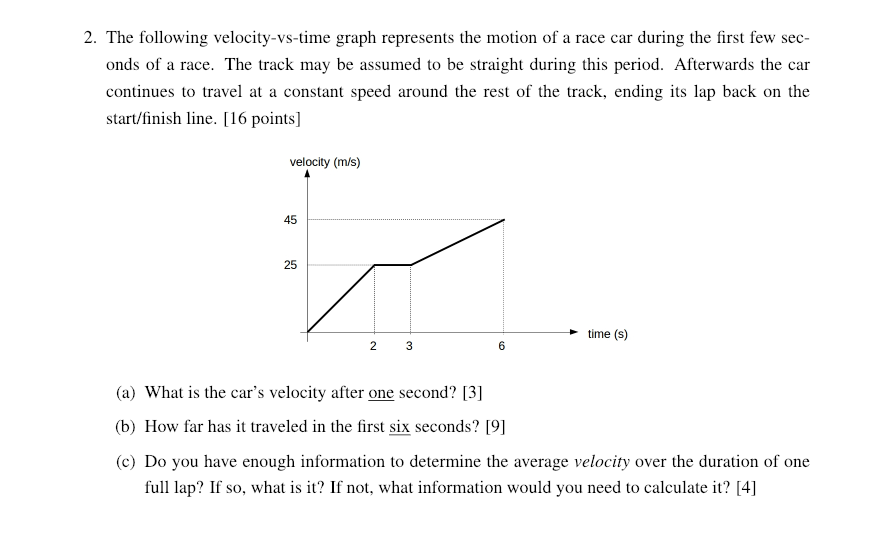2. The following velocity-vs-time graph represents the motion of a race car during the first few sec- onds of a race. The track may be assumed to be straight during this period. Afterwards the car continues to travel at a constant speed around the rest of the track, ending its lap back on the start/finish line. [16 points] velocity (m/s) 45 25 -time (S) 6 (a) What is the car's velocity after one second?  (b) How far has it...

Free Homework App